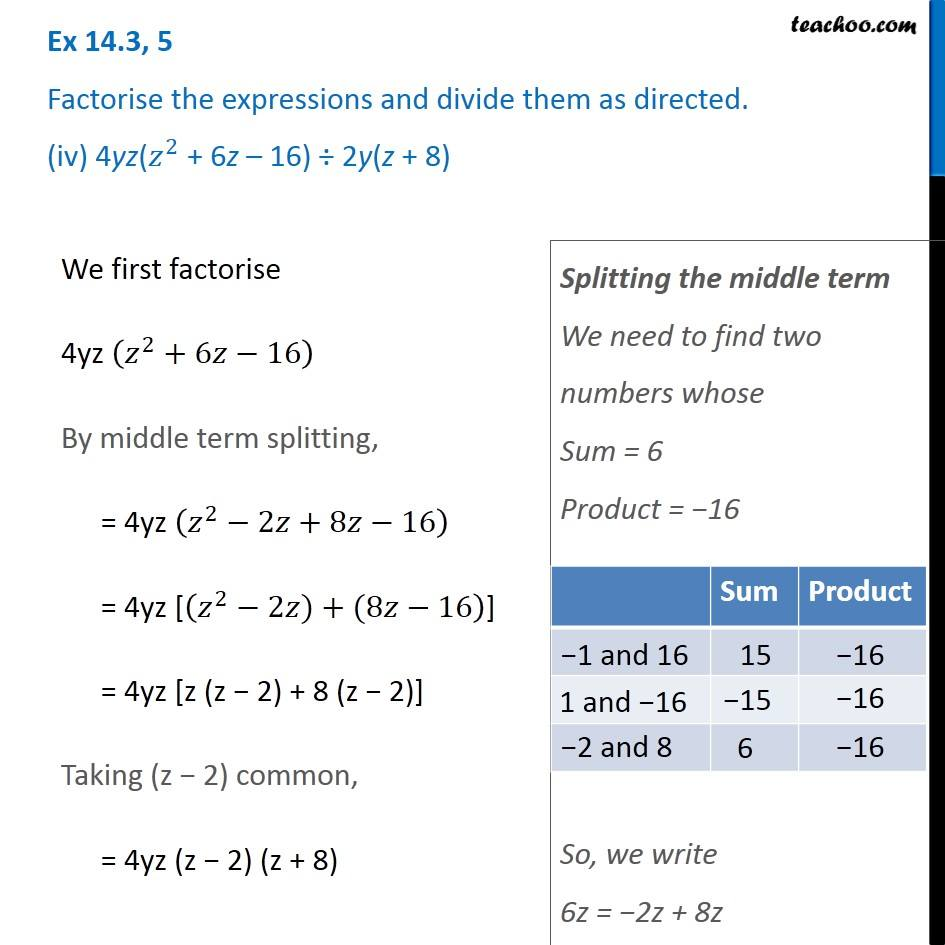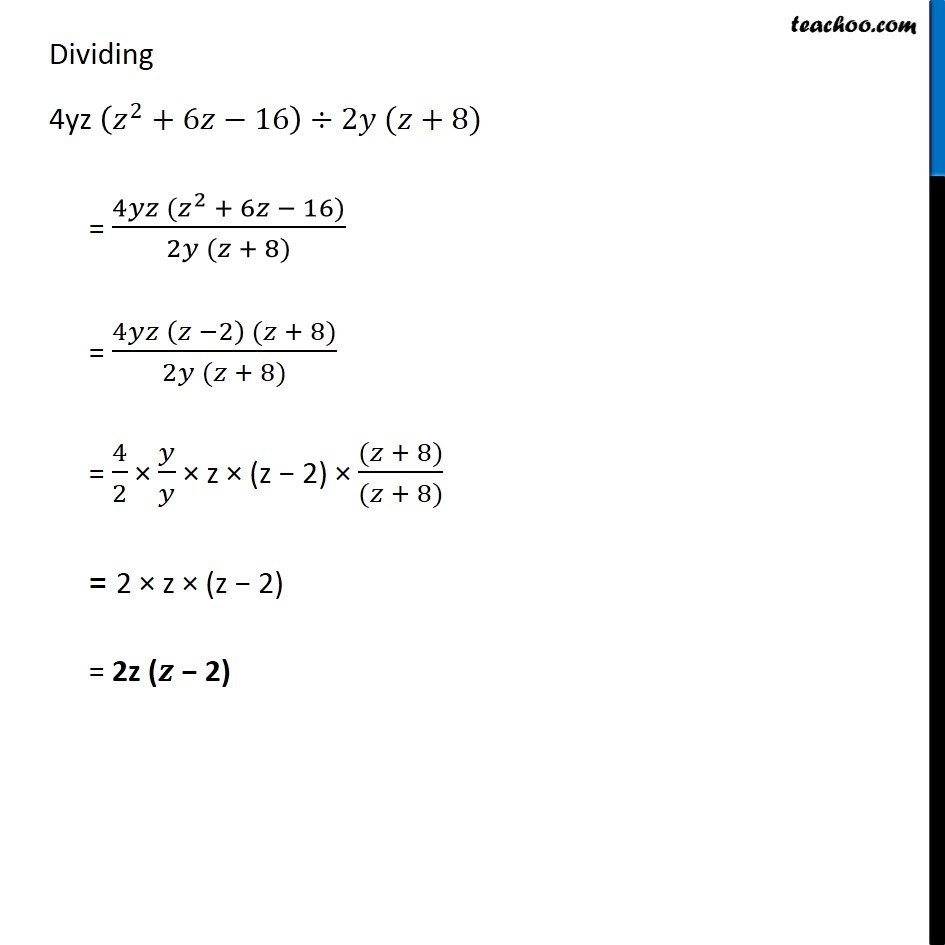Dividing two polynomials

Chapter 14 Class 8 Factorisation
Concept wiseIntroducing your new favourite teacher - Teachoo Black, at only ₹83 per month

### Transcript

Ex 14.3, 5 Factorise the expressions and divide them as directed. (iv) 4yz(𝑧^2 + 6z – 16) ÷ 2y(z + 8) We first factorise 4yz (𝑧^2+6𝑧−16) By middle term splitting, = 4yz (𝑧^2−2𝑧+8𝑧−16) = 4yz [(𝑧^2−2𝑧)+(8𝑧−16)] = 4yz [z (z − 2) + 8 (z − 2)] Taking (z − 2) common, = 4yz (z − 2) (z + 8) Splitting the middle term We need to find two numbers whose Sum = 6 Product = −16 So, we write 6z = −2z + 8z Dividing 4yz (𝑧^2+6𝑧−16)÷2𝑦 (𝑧+8) = (4𝑦𝑧 (𝑧^2 + 6𝑧 − 16))/(2𝑦 (𝑧 + 8)) = (4𝑦𝑧 (𝑧 −2) (𝑧 + 8))/(2𝑦 (𝑧 + 8)) = 4/2 × 𝑦/𝑦 × z × (z − 2) × ((𝑧 + 8))/((𝑧 + 8)) = 2 × z × (z − 2) = 2z (𝒛 − 2)